• maxpool2d参数
万次阅读
更多相关内容
• MaxPool2d 参数介绍 kernel_size ：表示做最大池化的窗口大小，可以是单个值，也可以是tuple元组 stride ：步长，可以是单个值，也可以是tuple元组 padding ：填充，可以是单个值，也可以是tuple元组 dilation ：...

在神经网络中 池化层是比较重要的，是提取重要信息的操作，可以去掉不重要的信息，减少计算开销。下面我们来介绍
MaxPool2d的使用方法。

API官网文档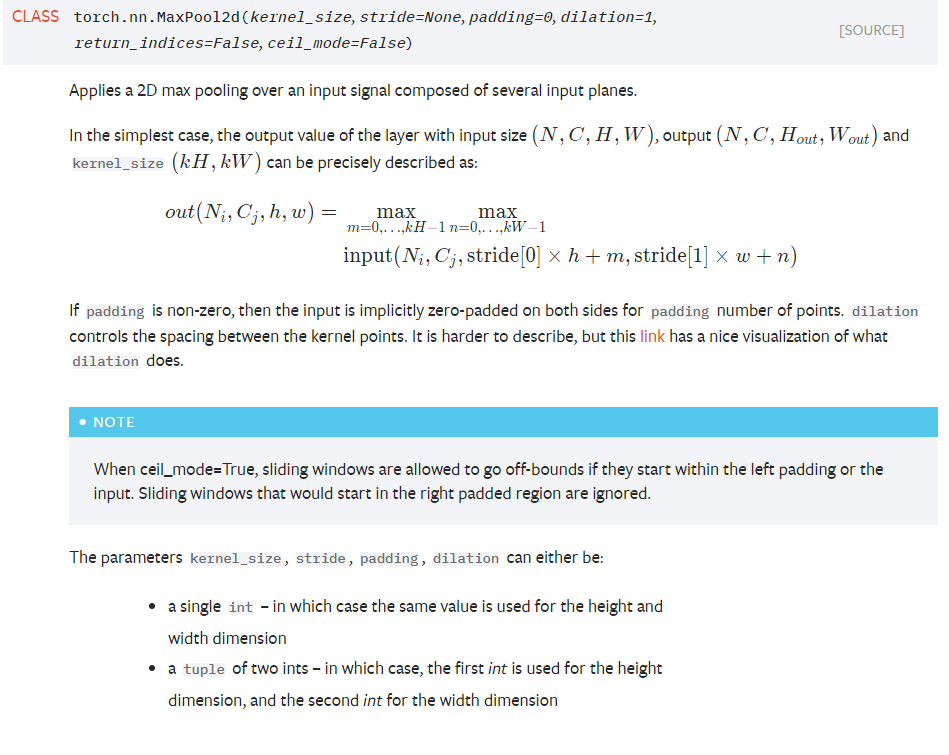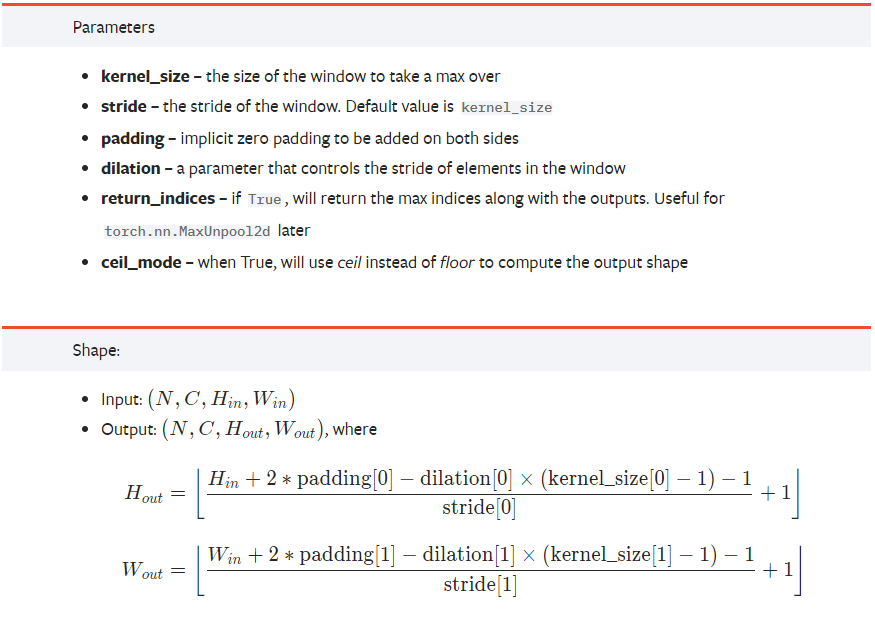## MaxPool2d 参数介绍

• kernel_size ：表示做最大池化的窗口大小，可以是单个值，也可以是tuple元组
• stride ：步长，可以是单个值，也可以是tuple元组
• dilation ：控制窗口中元素步幅
• return_indices ：布尔类型，返回最大值位置索引
• ceil_mode ：布尔类型，为True，用向上取整的方法，计算输出形状；默认是向下取整。

### kernel_size 的详解

注意这里的 kernel_size 跟卷积核不是一个东西。 kernel_size 可以看做是一个滑动窗口，这个窗口的大小由自己指定，如果输入是单个值，例如 3 ，那么窗口的大小就是 3 × 3 3 ，还可以输入元组，例如 (3, 2) ，那么窗口大小就是 3 × 2。
最大池化的方法就是取这个窗口覆盖元素中的最大值

### stride 的详解

上一个参数我们确定了滑动窗口的大小，现在我们来确定这个窗口如何进行滑动。如果不指定这个参数，那么默认步长跟最大池化窗口大小一致。如果指定了参数，那么将按照我们指定的参数进行滑动。例如 stride=(2,3) ， 那么窗口将每次向右滑动三个元素位置，或者向下滑动两个元素位置

### dilation 的详解

空洞卷积，默认 dilation=1，如果kernel_size =3，那么卷积核就是33的框。如果dilation = 2，kernel_size =3，那么每列数据与每列数据中间再加一列空洞，那么卷积核就变成55的框。

### return_indices 的详解

这是个布尔类型值，表示返回值中是否包含最大值位置的索引。注意这个最大值指的是在所有窗口中产生的最大值，如果窗口产生的最大值总共有5个，就会有5个返回值。

### ceil_mode 的详解

这个也是布尔类型值，它决定的是在计算输出结果形状的时候，是使用向上取整还是向下取整。怎么计算输出形状，下面会讲到。一看就知道了。

### 最大池化层输出形状计算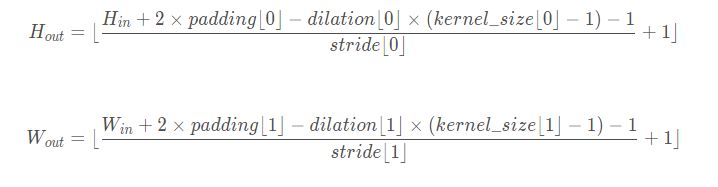看到向下取整的符号了吗？这个就是由 ceil_mode 控制的。

## 参数示例介绍

### 验证 kernel_size 参数

import torch
import torch.nn as nn

# 仅定义一个 3x3 的池化层窗口
m = nn.MaxPool2d(kernel_size=(3, 3))

# 定义输入
# 四个参数分别表示 (batch_size, C_in, H_in, W_in)
# 分别对应，批处理大小，输入通道数，图像高度（像素），图像宽度（像素）
# 为了简化表示，我们只模拟单张图片输入，单通道图片，图片大小是6x6
input = torch.randn(1, 1, 6, 6)

print(input)

output = m(input)

print(output)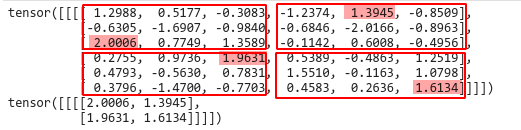第一个tensor是我们的输入数据 1 × 1 × 6 × 6 ，我们画红线的区域就是我们设置的窗口大小 3 × 3 ，背景色为红色的值，为该区域的最大值。

第二个tensor就是我们最大池化后的结果，跟我们标注的一模一样。

### 验证一下 stride 参数

import torch
import torch.nn as nn

# 仅定义一个 3x3 的池化层窗口
m = nn.MaxPool2d(kernel_size=(3, 3), stride=(2, 2))

# 定义输入
# 四个参数分别表示 (batch_size, C_in, H_in, W_in)
# 分别对应，批处理大小，输入通道数，图像高度（像素），图像宽度（像素）
# 为了简化表示，我们只模拟单张图片输入，单通道图片，图片大小是6x6
input = torch.randn(1, 1, 6, 6)

print(input)

output = m(input)

print(output)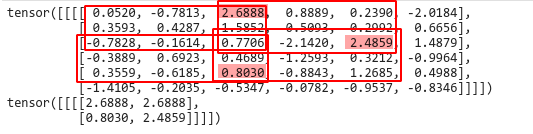红色的还是我们的窗口，但是我们的步长变为了2，可以看到第一个窗口和向右滑动后的窗口，他们的最大值刚好是重叠的部分都是2.688，向下滑动之后，最大值是0.8030，再次向右滑动，最大值是2.4859。

可以看到我们在滑动的时候省略了部分数值，因为剩下的数据不够一次滑动了，于是我们将他们丢弃了。

其实最后图片的宽度和高度还可以通过上面两个公式来计算，我们公式中用的是向下取整，因此我们丢弃了不足的数据。现在我们试试向上取整。

### 利用 ceil_mode 参数向上取整

import torch
import torch.nn as nn

# 仅定义一个 3x3 的池化层窗口
m = nn.MaxPool2d(kernel_size=(3, 3), stride=(2, 2), ceil_mode=True)

# 定义输入
# 四个参数分别表示 (batch_size, C_in, H_in, W_in)
# 分别对应，批处理大小，输入通道数，图像高度（像素），图像宽度（像素）
# 为了简化表示，我们只模拟单张图片输入，单通道图片，图片大小是6x6
input = torch.randn(1, 1, 6, 6)

print(input)

output = m(input)

print('\n\n\n\n\n')

print(output)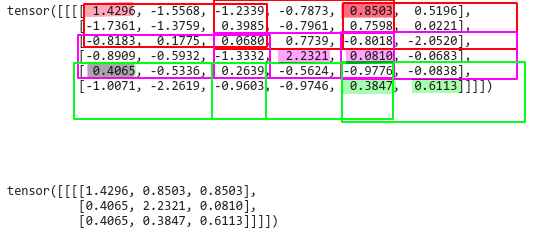从结果可以看出，输出的size由原来的 2 × 2 变成了现在的 3 × 3。这就是向上取整的结果。为什么会出现这样的结果呢？

这看起来像是我们对输入进行了填充，但是这个填充值不会参与到计算最大值中

import torch
import torch.nn as nn

# 仅定义一个 3x3 的池化层窗口
m = nn.MaxPool2d(kernel_size=(3, 3), stride=(3, 3), padding=(1, 1))

# 定义输入
# 四个参数分别表示 (batch_size, C_in, H_in, W_in)
# 分别对应，批处理大小，输入通道数，图像高度（像素），图像宽度（像素）
# 为了简化表示，我们只模拟单张图片输入，单通道图片，图片大小是6x6
input = torch.randn(1, 1, 6, 6)

print(input)

output = m(input)

print('\n\n')

print(output)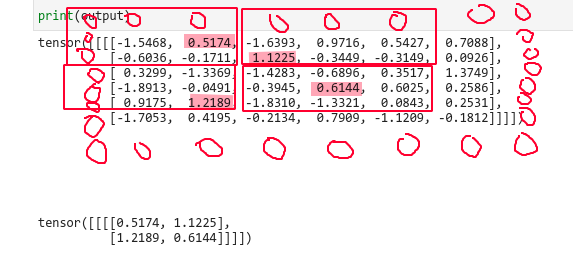我们对周围填充了一圈0，我们滑动窗口的范围就变化了，这就是填充的作用。

但是有一点需要注意，就是即使我们填充了0，这个0也不会被选为最大值。例如上图的左上角四个数据，如果我们全部变为负数，结果是-0.1711，而不会是我们填充的0值，这一点要注意。

### 验证 return_indices 参数

import torch
import torch.nn as nn

# 仅定义一个 3x3 的池化层窗口
m = nn.MaxPool2d(kernel_size=(3, 3), return_indices=True)

# 定义输入
# 四个参数分别表示 (batch_size, C_in, H_in, W_in)
# 分别对应，批处理大小，输入通道数，图像高度（像素），图像宽度（像素）
# 为了简化表示，我们只模拟单张图片输入，单通道图片，图片大小是6x6
input = torch.randn(1, 1, 6, 6)

print(input)

output = m(input)

print(output)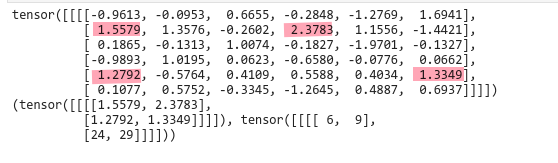### 验证 dilation 参数


python
import torch
import torch.nn as nn

# 仅定义一个 3x3 的池化层窗口
m = nn.MaxPool2d(kernel_size=(3, 3),dilation=2)

# 定义输入
# 四个参数分别表示 (batch_size, C_in, H_in, W_in)
# 分别对应，批处理大小，输入通道数，图像高度（像素），图像宽度（像素）
# 为了简化表示，我们只模拟单张图片输入，单通道图片，图片大小是6x6
input = torch.randn(1, 1, 6, 6)

print(input)

output = m(input)

print(output)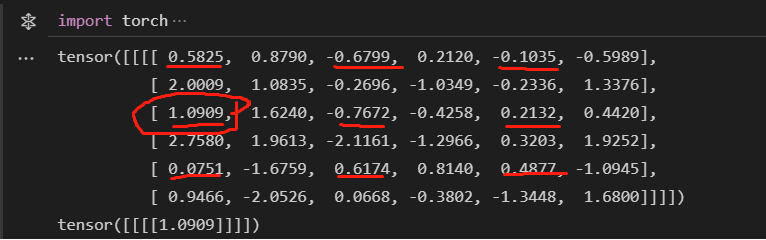参考文档: https://blog.csdn.net/weixin_38481963/article/details/109962715

展开全文pytorch 深度学习 神经网络
• class torch.nn.MaxPool2d(kernel_size, stride=None, padding=0, dilation=1, return_indices=False, ceil_mode=False) 如下是MaxPool2d的解释： class MaxPool2d(_MaxPoolNd): r"""Applies a 2D max pooling over...

日常学习，给自己挖坑，and造轮子

class torch.nn.MaxPool2d(kernel_size, stride=None, padding=0, dilation=1, return_indices=False, ceil_mode=False)
如下是MaxPool2d的解释：

class MaxPool2d(_MaxPoolNd):
r"""Applies a 2D max pooling over an input signal composed of several input
planes.

In the simplest case, the output value of the layer with input size :math:(N, C, H, W),output :math:(N, C, H_{out}, W_{out}) and :attr:kernel_size :math:(kH, kW)can be precisely described as:

.. math::
\begin{aligned}
out(N_i, C_j, h, w) ={} & \max_{m=0, \ldots, kH-1} \max_{n=0, \ldots, kW-1} \\
& \text{input}(N_i, C_j, \text{stride} \times h + m,
\text{stride} \times w + n)
\end{aligned}

If :attr:padding is non-zero, then the input is implicitly zero-padded on both sides
for :attr:padding number of points. :attr:dilation controls the spacing between the kernel points.
It is harder to describe, but this link_ has a nice visualization of what :attr:dilation does.

The parameters :attr:kernel_size, :attr:stride, :attr:padding, :attr:dilation can either be:

- a single int -- in which case the same value is used for the height and width dimension
- a tuple of two ints -- in which case, the first int is used for the height dimension, and the second int for the width dimension

Args:
kernel_size: the size of the window to take a max over
stride: the stride of the window. Default value is :attr:kernel_size
dilation: a parameter that controls the stride of elements in the window
return_indices: if True, will return the max indices along with the outputs.
Useful for :class:torch.nn.MaxUnpool2d later
ceil_mode: when True, will use ceil instead of floor to compute the output shape

Shape:
- Input: :math:(N, C, H_{in}, W_{in})
- Output: :math:(N, C, H_{out}, W_{out}), where

.. math::
H_{out} = \left\lfloor\frac{H_{in} + 2 * \text{padding} - \text{dilation}
\times (\text{kernel\_size} - 1) - 1}{\text{stride}} + 1\right\rfloor

.. math::
W_{out} = \left\lfloor\frac{W_{in} + 2 * \text{padding} - \text{dilation}
\times (\text{kernel\_size} - 1) - 1}{\text{stride}} + 1\right\rfloor

Examples::

>>> # pool of square window of size=3, stride=2
>>> m = nn.MaxPool2d(3, stride=2)
>>> # pool of non-square window
>>> m = nn.MaxPool2d((3, 2), stride=(2, 1))
>>> input = torch.randn(20, 16, 50, 32)
>>> output = m(input)

"""

def forward(self, input):
return F.max_pool2d(input, self.kernel_size, self.stride,
self.return_indices)



大致解释为：
在由多个输入通道组成的输入信号上应用2D max池。

在最简单的情况下，具有输入大小的层的输出值：(N, C, H, W)
输出：(N, C, H_{out}, W_{out})kernel_size，和 (kH, kW)可以准确地描述为：

out(N_i, C_j, h, w) ={} & \max_{m=0, \ldots, kH-1} \max_{n=0, \ldots, kW-1} \\
& \text{input}(N_i, C_j, \text{stride} \times h + m,
\text{stride} \times w + n)


如果 padding 非零，那么输入的两边都隐式地被0填充；dilation用于控制内核点之间的距离
这里很好地展示了 diagration 的作用。

这些参数：kernel_sizestridepadding, ，dilation 可以为：
-单个int值–在这种情况下，高度和宽度标注使用相同的值
-两个整数组成的数组——在这种情况下，第一个int用于高度维度，第二个int表示宽度

参数：

kernel_size(int or tuple) ：max pooling的窗口大小
stride(int or tuple, optional)：max pooling的窗口移动的步长。默认值是kernel_size
padding(int or tuple, optional) ：输入的每一条边补充0的层数
dilation(int or tuple, optional)：一个控制窗口中元素步幅的参数
return_indices ：如果等于True，会返回输出最大值的序号，对于上采样操作会有帮助
ceil_mode ：如果等于True，计算输出信号大小的时候，会使用向上取整，代替默认的向下取整的操作

Shape:
- Input: :math:(N, C, H_{in}, W_{in})
- Output: :math:(N, C, H_{out}, W_{out}), where

.. math::
H_{out} = \left\lfloor\frac{H_{in} + 2 * \text{padding} - \text{dilation}
\times (\text{kernel\_size} - 1) - 1}{\text{stride}} + 1\right\rfloor

.. math::
W_{out} = \left\lfloor\frac{W_{in} + 2 * \text{padding} - \text{dilation}
\times (\text{kernel\_size} - 1) - 1}{\text{stride}} + 1\right\rfloor

Examples::

>>> # pool of square window of size=3, stride=2
>>> m = nn.MaxPool2d(3, stride=2)
>>> # pool of non-square window
>>> m = nn.MaxPool2d((3, 2), stride=(2, 1))
>>> input = torch.randn(20, 16, 50, 32)
>>> output = m(input)
`
展开全文python
•展开全文• ## MaxPool2d()参数解释

万次阅读 2020-07-02 17:02:57
class torch.nn.MaxPool2d(kernel_size, stride=None, padding=0, dilation=1, return_indices=False, ceil_mode=False) 对于输入信号的输入通道，提供2维最大池化（max pooling）操作 如果输入的大小是(N,C,H,W)...
• class torch.nn.MaxPool2d( kernel_size, stride=None, padding=0, dilation=1, return_indices=False, ceil_mode=False) 2 参数详解 kernel_size 最大池化窗口的大小，类型为int 或者...pytorch python
• tf.keras.layers.MaxPool2D( pool_size=(2, 2), strides=None, padding='valid', data_format=None, **kwargs ) 这个函数给出了4个关键字参数，都是有 默认值的，这意味着你如果一个参数都不给，也是可以的。 ...tensorflow python
• ## torch.nn.MaxPool2d详解

万次阅读 多人点赞 2020-11-22 20:18:38
之后我们验证一下 stride 参数： import torch import torch.nn as nn # 仅定义一个 3x3 的池化层窗口 m = nn.MaxPool2d(kernel_size=(3, 3), stride=(2, 2)) # 定义输入 # 四个参数分别表示 (batch_size, C_in, H_...深度学习
• torch.nn.MaxPool2d和torch.nn.functional.max_pool2d，在pytorch构建模型中，都可以作为最大池化层的引入，但前者为类模块，后者为函数，在使用上存在不同。 1. torch.nn.functional.max_pool2d pytorch中的函数，...pytorch
• Pooling layers池化层中有许多函数，其中使用较多的就是最大池化MaxPool2d，本文结合代码简单讲解一下MaxPool2d的使用。神经网络 python 人工智能 深度学习
• 官方文档 作用 计算最大值池化 定义 def __init__(self, pool_size=(2, 2), strides=None, padding='valid', data_format=None, **kwargs) 输入 如果da...pytorch
• Pytorch迟化层Maxpool2d中ceil_mode参数 当ceil_mode = true时，将保存不足为kernel_size大小的数据保存，自动补足NAN至kernel_size大小； 当ceil_mode = False时，剩余数据不足kernel_size大小时，直接舍弃。 例如...python
• 相关文章 Pytorch学习笔记(一):torch.cat()模块的详解 文章目录1.函数语法格式和作用2.参数解释3.... 1.函数语法格式和作用 ...MaxPool2d(kernel_size, stride=None, padding=0, dilation=1, return_python 深度学习 人工智能 pytorch
• torch.nn.MaxPool2d 功能： MaxPool 最大池化层，池化层在卷积神经网络中的作用在于特征融合和降维。池化也是一种类似的卷积操作，只是池化层的所有参数都是超参数，是学习不到的。 作用： maxpooling有局部不变性...
• ## torch.nn.MaxPool2d

万次阅读 多人点赞 2020-07-28 15:57:52
• 第1章 关于1维MaxPool1d、2维MaxPool2d、3维MaxPool3d的说明 第2章MaxPool2d详解 2.1 功能说明 2.2MaxPool2d的本质 2.3 类原型 2.4 参数说明 前言： 本文是深度学习框架 pytorch 的API ： torch.nn.MaxPool2d...pytorch 深度学习
• 1.MaxPool2d 假设现在有一个4×4的图片，以及一个3 ×3的卷积核，在没有padding的情况下，逐列逐行扫描, 即步长，stride = 1。扫描过程如下，就是取每一个核中的最大值 代码实现： 假设batch = 2，channel=1， 即有...卷积 深度学习 神经网络
• 在上一节中我们详细的阐述了Conv2d的计算原理，今天我们来讲述下Pytorch中其他比较常见的操作！...在pytorch中使用Pooling操作来实现采样，常见的pool操作包含Max_pool，Avg_poolMax_pool x = t.rand(1,3,7,7...Pytorch
• class torch.nn.MaxPool2d(kernel_size, stride=None, padding=0, dilation=1, return_indices=False, ceil_mode=False) kernel_size(int or tuple) - max pooling的窗口大小 stride(int or tuple, optional) - max...
• 使用最大值操作的池化层被称之为最大池化层（max pooling），使用平均值操作的池化层称之为平均池化层（average pooling），总的来说，池化层的作用是可以压缩数据和参数的量， 减小过拟合。 如下
• 1.tf.nn.maxpool2d()函数介绍 tf.nn.max_pool2d(input, ksize, strides, padding, data_format='NHWC', name=None) 参数说明： Args input A 4-DTensorof the format specified bydata_format. ...深度学习
• MaxPool1d torch.nn.MaxPool1d(kernel_size, stride=None, padding=0, dilation=1, return_indices=False, ceil_mode=False) 输入size为(N,C,L)，在L维进行池化 参数： kernel_size – 池化窗口大小 stride – ...
• 1. nn.Conv2d nn.Conv2d 输入信号的形式为(N, Cin, H, W), N表示batch size，Cin​表示channel个数，H，W分别表示特征图的高和宽。...参数说明： stride(步长)：控制cross-correlation的步长，可...
• 1.应用 import torch import torch.nn as nn m = nn.MaxPool2d(2) ...CLASS torch.nn.MaxPool2d(kernel_size: Union[T, Tuple[T, ...]], stride: Optional[Union[T, Tuple[T, ...]]] = None, padding: Unionpython
• ## MaxPool2d

千次阅读 2022-02-15 20:54:33
(kernel_size, stride=None, padding=0, dilation=1,...) kernel_size：卷积核大小 stride：官方定义stride=传入的stride参数或者stride=kernel_size深度学习 计算机视觉 目标检测
• MaxPool2d案例 1.34.1.2. AvgPool2d案例 1.34.2. F.interpolate案例（上采样） 1.34.3.ReLU案例 1.34.PyTorch Down/up sample (pytorch上下采样) 参考地址：https://cloud.tencent.com/developer/article/1542468 ...
• 卷积神经网络中nn.Conv2d()和nn.MaxPool2d() 卷积神经网络之Pythorch实现： nn.Conv2d()就是PyTorch中的卷积模块 参数列表 参数 作用 in_channels 输入数据体的深度 out_channels 输出数 据体的深度 ...分类 神经网络
• # def max_pool2d(inputs, # kernel_size, # stride=2, # padding='VALID', # data_format=DATA_FORMAT_NHWC, # outputs_collections......